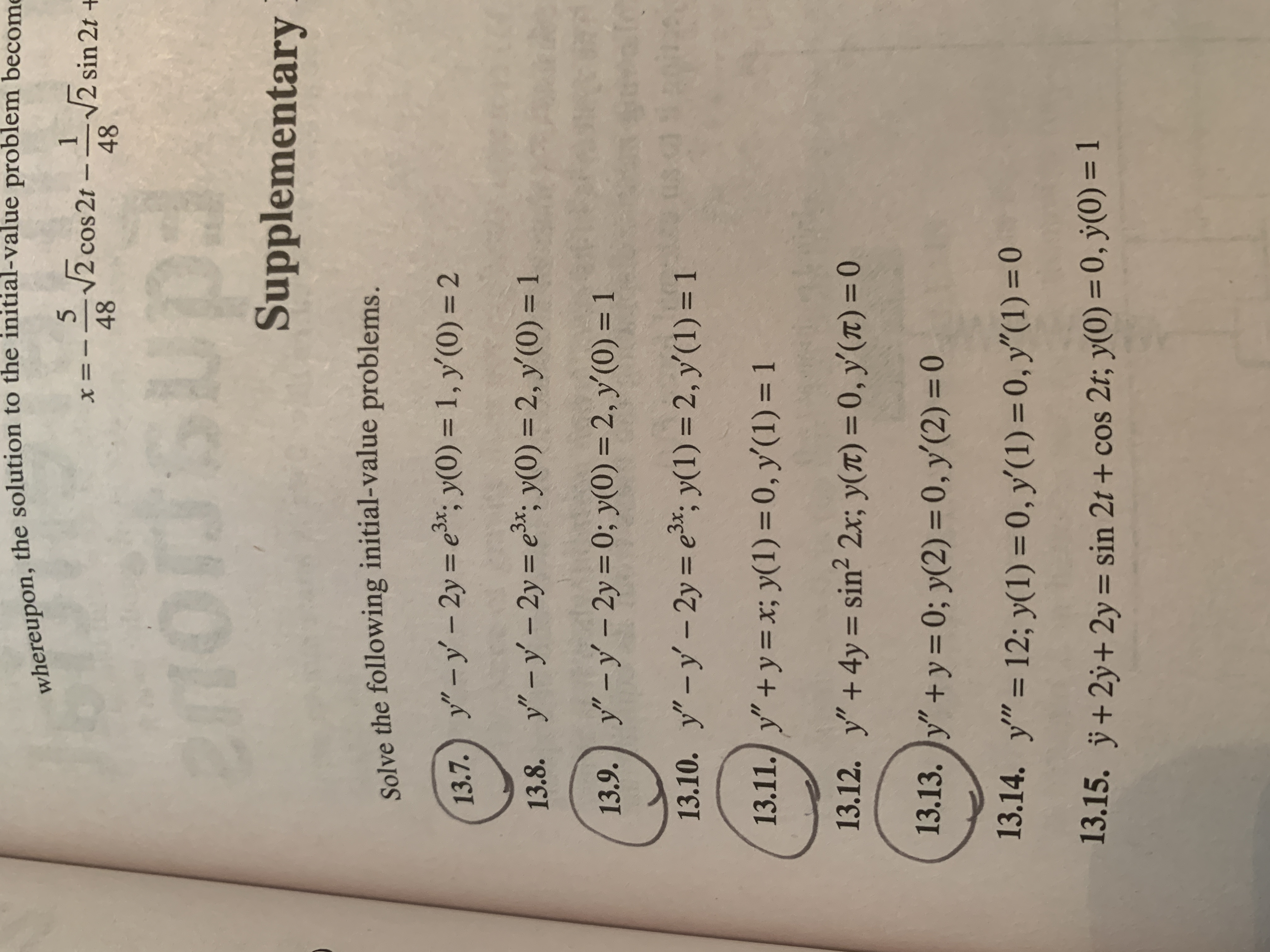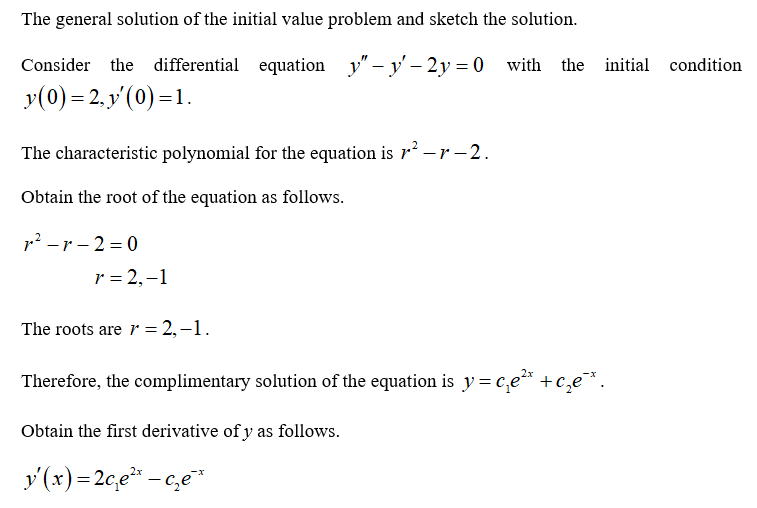# whereupon, the solution to the initial-value problem become2 cos 2t-2 sin 21 +4848SupplementarySolve the following initial-value problems.13.7.) y"-y' - 2y = e*; y(0) = 1, y'(0) = 2%3Dy" -y - 2y = e*; y(0) = 2, y'(0) = 113.8.%3D13.9.) y"-y - 2y = 0; y(0) = 2, y'(0) = 113.10. y"-y-2y = e3*, y(1) = 2, y'(1) = 113.11. y"+y = x; y(1) = 0, y'(1) = 113.12. y"+ 4y = sin? 2x; y(T) = 0, y(T) = 0%3D%3D13.13. )y" + y=0; y(2) = 0, y'(2) = 0%3D13.14.y" = 12; y(1) = 0, y'(1) = 0, y"(1) = 0%3D%3D13.15. ÿ+2ÿ+2y = sin 2t + cos 2t; y(0) = 0, ý(0) = 1%3D%3D

Question
1 views

Question #13.9 from the image. Differential Equationshelp_outlineImage Transcriptionclosewhereupon, the solution to the initial-value problem become 2 cos 2t-2 sin 21 + 48 48 Supplementary Solve the following initial-value problems. 13.7.) y"-y' - 2y = e*; y(0) = 1, y'(0) = 2 %3D y" -y - 2y = e*; y(0) = 2, y'(0) = 1 13.8. %3D 13.9.) y"-y - 2y = 0; y(0) = 2, y'(0) = 1 13.10. y"-y-2y = e3*, y(1) = 2, y'(1) = 1 13.11. y"+y = x; y(1) = 0, y'(1) = 1 13.12. y"+ 4y = sin? 2x; y(T) = 0, y(T) = 0 %3D %3D 13.13. )y" + y=0; y(2) = 0, y'(2) = 0 %3D 13.14. y" = 12; y(1) = 0, y'(1) = 0, y"(1) = 0 %3D %3D 13.15. ÿ+2ÿ+2y = sin 2t + cos 2t; y(0) = 0, ý(0) = 1 %3D %3D fullscreen
check_circle

Step 1...

### Want to see the full answer?

See Solution

#### Want to see this answer and more?

Solutions are written by subject experts who are available 24/7. Questions are typically answered within 1 hour.*

See Solution
*Response times may vary by subject and question.
Tagged in